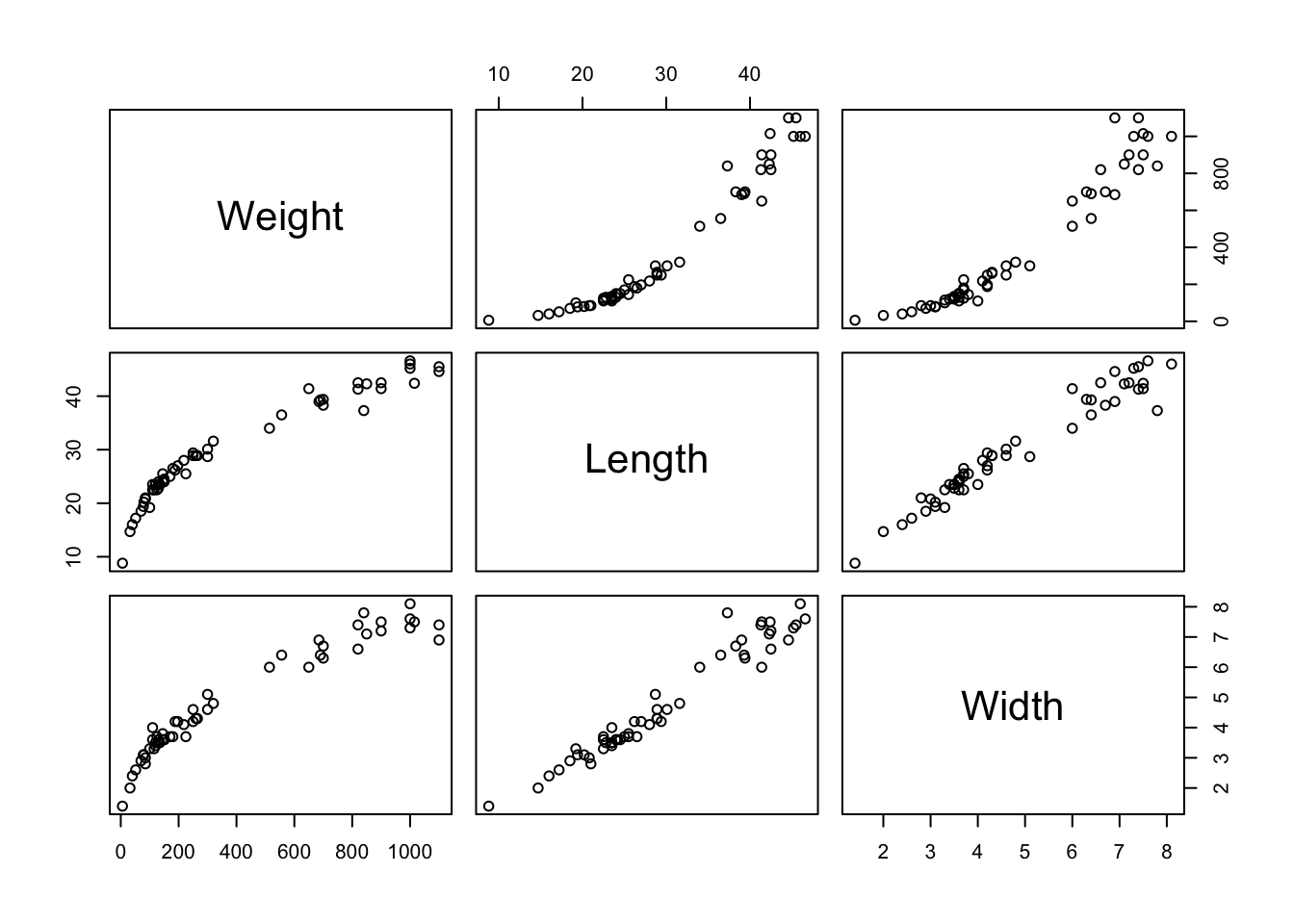We have information on perch- a type of fish- caught in a lake in Finland. For each of the 56 fish caught we have data on their weight (in grams), length (in cm), and width (in cm). Create a model using the variables collected to predict the weight of perch causing at Lake Laengelmavesi in Finland.

First, let’s calculate the correlation between each of the terms using a correlation matrix as well as a scatterplot matrix.

``cor(Perch[, c(2:4)])``
``````##           Weight    Length     Width
## Weight 1.0000000 0.9595061 0.9642244
## Length 0.9595061 1.0000000 0.9751074
## Width  0.9642244 0.9751074 1.0000000``````
``plot(Perch[, c(2:4)])``It appears that both width and length are highly correlated with weight; however, when we look at the scatterplot matrix the relationships do not appear to be linear.

``````p1 <- ggplot(Perch) + geom_point(aes(x = Length, y = Weight)) +
labs(x = "Length", y = "Weight", title = "Scatterplot: Weight vs Length")

p2 <- ggplot(Perch) + geom_point(aes(x = Length^2, y = Weight)) +
labs(x = "Length^2", y = "Weight", title = "Scatterplot: Weight vs Length^2")

p3 <- ggplot(Perch) + geom_point(aes(x = Width, y = Weight)) +
labs(x = "Width", y = "Weight", title = "Scatterplot: Weight vs Width")

p4 <- ggplot(Perch) + geom_point(aes(x = Width^2, y = Weight)) +
labs(x = "Width^2", y = "Weight", title = "Scatterplot: Weight vs Width^2")

grid.arrange(p1,p2,p3,p4)``````